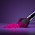Solving for a firm's profits under monopolistic and perfectly competitive conditions - FreeEconHelp.com, Learning Economics... Solved!

## 9/24/11

Here is another question recently received about mathematically solving for a firms equilibrium price, quantity, and profits under two different market structures: a monopoly, and perfect competition.

A firm faces the inverse demand curve: P = 300 – 0.5*Q
Which has the corresponding marginal revenue function: MR = 300 – 1*Q
Where:
Q is monthly production and
P is price, measured in \$/unit
The firm also has a total cost (TC) function: TC = 4,000 + 45Q
Assuming the firm maximizes profits, answer the following:
1. Assuming the firm operates as a monopolist, calculate the (i) price, (ii) quantity and (iii) profit. Graph and show the equilibrium price and quantity.
2. Assuming perfect competition, calculate the (i) price, (ii) quantity, and (iii)
profit? Show the equilibrium price and quantity on the same graph as 1.

This type of problem is relatively straight forward.  We are given a demand function, a marginal revenue function, and a total cost function.  Remember that when maximizing profit we want to set marginal cost to marginal benefit (MC=MB) and in this particular problem, the MB is going to be MR (marginal revenue).  So let’s figure out what marginal cost is going to be.

If you know calculus, then you can just take the derivative of total cost with respect to Q.  If you do not know calculus, then just find the slope of the total cost line.  Either way you get a marginal cost of 45.

Then you must set MC=MR so 45 = 300 –Q.

Add Q to both sides, and subtract 45 from both sides to get Q = 255.

So our equilibrium quantity will be 255, and we can plug this into our inverse demand function to get:
P = 300-.5Q =300 - .5(255) = 172.5

Multiply our equilibrium P by equilibrium Q to get total revenue: 172.5*255 = 43,987.5.

We can also plug our equilibrium quantity into the total cost function to get total cost.  TC = 4,000 + 45 * 255 = 11,475.

Total profit is going to be equal to total revenue minus total cost, so our total profit will be 43,987.5 – 11,475 = 32,512.5.

For perfect competition we set marginal cost to price (MC=P), so the price will be \$45.  We can put that price into our inverse demand function and solve for Q:  45= 300 - .5*Q

Add .5 Q to both sides, subtract 45 from both sides, then multiply both sides by 2 to get:

Q = 510

So under perfect competition, equilibrium price is \$45, and equilibrium quantity is 510.  This makes sense, because our intuition tells us the perfectly competitive markets will provide products at a lower price, and also provide more of the good to the market.

1.2.3.